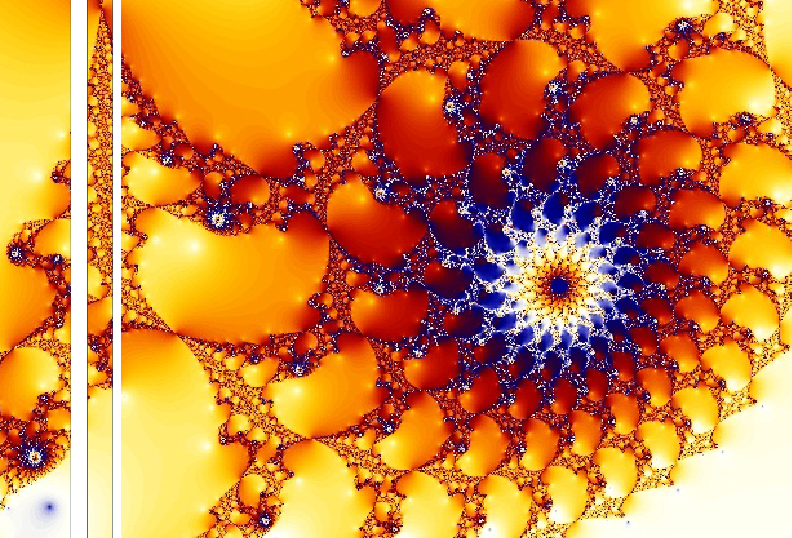Univerza na Primorskem Fakulteta za matematiko, naravoslovje in informacijske tehnologijeSI | EN# Raziskovalni matematični seminar - Arhiv

 2021 2020 2019 2018 2017 2016 2015 2014 2013 2012 2011 2010
 1 2 3 4 5 6 7 8 9 10 11 12
Datum in ura / Date and time: 31.3.14
(10:00-11:00)
Predavalnica / Location: FAMNIT-SEMIN
Predavatelj / Lecturer: Irina Cristea (University of Nova Gorica, Slovenia)
Naslov / Title: Some combinatorial aspects of the connections between hypergroups and fuzzy sets

Datum in ura / Date and time: 24.3.14
(10:00-11:00)
Predavalnica / Location: FAMNIT-SEMIN
Predavatelj / Lecturer: Martin Milanič
Naslov / Title: Counterexamples to Orlin's conjecture on equistable graphs
Vsebina / Abstract:

A stable set in a graph is a set of pairwise non-adjacent vertices, and a stable set is maximal if it is not contained in any other stable set. Equistable graphs are graphs admitting positive weights on vertices such that a subset of vertices is a maximal stable set if and only if it is of total weight 1. General partition graphs are the intersection graphs of set systems over a finite ground set S such that every maximal stable set of the graph corresponds to a partition of S. It is known that general partition graphs are exactly the graphs such that every edge is contained in a strong clique (a clique is strong if it intersects all maximal stable sets).

No combinatorial characterization of equistable graphs is known. In 2009, Orlin proved that every general partition graph is equistable, and conjectured that the converse holds as well. Orlin's conjecture has been verified for several graph classes, including line graphs, simplicial graphs, very  well-covered graps, chordal graphs, series-parallel graphs, AT-free graphs, EPT graphs, and certain graph products. Orlin's conjecture, if true, would imply another conjecture on equistable graphs due to Mahadev, Peled, and Sun from 1994, stating that every equistable graph is strongly equistable. A conjecture that would follow from Orlin's conjecture and would imply the conjecture by Mahadev, Peled, and Sun was posed in 2011 by Miklavič and Milanič in 2011, and states that every equistable graph has a strong clique.

In the talk, I will present an infinite family of counterexamples to Orlin's conjecture. The family contains equistable graphs not having any strong cliques, and hence also disproves the conjecture by Miklavič and Milanič.

The results were obtained jointly with Stéphan Thomassé and Nicolas Trotignon, during a recent visit of the speaker at ENS Lyon.

DOWNLOAD SLIDES!

Datum in ura / Date and time: 17.3.14
(10:00-11:00)
Predavalnica / Location: FAMNIT-SEMIN
Predavatelj / Lecturer: Paweł Petecki
Naslov / Title: On cyclic Hamiltonian decompositions of complete k-uniform hypergraphs
Vsebina / Abstract:

A decomposition $\mathcal{C}=\{ C_1,C_2,...,C_h\}$ of the complete $k$-uniform hypergraph $K^k_n$ of order $n$ is called {\em cyclic Hamiltonian} if each $C_i\in \mathcal{C}$, $i\in\{1,2,\ldots,h\}$,  is a Hamiltonian cycle in $K^k_n$ and there exists a permutation $\sigma$ of the vertex set of $K^k_n$ having exactly one cycle in its cycle decomposition such that for every cycle $C_i\in\mathcal{C}$ its set of edges coincides with an orbit of $\langle\sigma\rangle$ when acting on the edge set of $K^k_n$.  In this paper it is shown that $K_n^k$ admits a cyclic Hamiltonian decomposition if and only if $n$ and $k$ are relatively prime and $\lambda=\min\{d>1:\ d|n\}>\frac nk$.

Datum in ura / Date and time: 10.3.14
(10:00-11:00)
Predavalnica / Location: FAMNIT-SEMIN
Predavatelj / Lecturer: Nina Chiarelli
Naslov / Title: On a class of graphs between threshold and total domishold graphs
Vsebina / Abstract:

Download abstract!